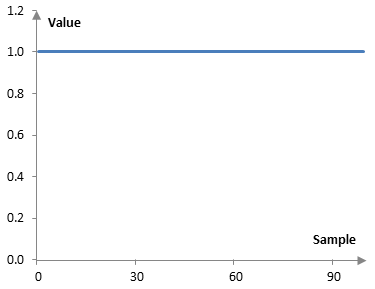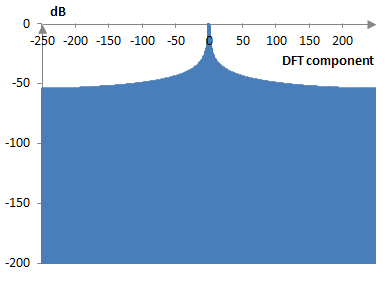# Rectangular window

The rectangular window of length N, defined for k = 0, 1, …, N – 1 is given by the formula

$$a(k)=1$$

The window has no effect when applied to a filter or a signal. It is, however, a benchmark window, against which other windows are often compared. For example, a standard low pass filter with finite impulse response will have the shortest transition band with the rectangular window.

The following is a graph of the rectangular window (N = 100).## Measures for the rectangular window

The following is a discrete Fourier transform of 500 points of the rectangular window.The window measures are as follows.

 Coherent gain 1.0 Equivalent noise bandwidth 1.0 Processing gain 0.0 dB Scalloping loss -3.92 dB Worst case processing loss -3.92 dB Highest sidelobe level -13.3 dB Sidelobe falloff -6.0 dB / octave, -20 dB / decade Main lobe is -3 dB 0.88 bins Main lobe is -6 dB 1.20 bins Overlap correlation at 50% overlap 0.500 bins Amplitude flatness at 50% overlap 1.000 bins Overlap correlation at 75% overlap 0.752 bins Amplitude flatness at 75% overlap 1.000 bins

Window

### Filtered HTML

• Freelinking helps you easily create HTML links. Links take the form of [[indicator:target|Title]]. By default (no indicator): Click to view a local node.